Home | | Statistics 12th Std | StudentŌĆÖs t Distribution and Its Applications

# StudentŌĆÖs t Distribution and Its Applications

General Procedure for StudentŌĆÖs t Distribution and Its Applications, Properties, Procedure Steps, Example Solved Problems

STUDENTŌĆÖS t DISTRIBUTION AND ITS APPLICATIONS

## 1. StudentŌĆÖs t-distribution

If X~N(0,1) and Y~Žćn2 are independent random variables, thenis said to have t-distribution with n degrees of freedom. This can be denoted by tn.

Note 1: The degrees of freedom of t is the same as the degrees of freedom of the corresponding chi-square random variable.

Note 2: The t-distribution is used as the sampling distribution(s) of the statistics(s) defined based on random sample(s) drawn from normal population(s).

(i) If X1, X2 , ŌĆ”, Xn is a random sample drawn from N (╬╝, Žā2) population then(ii) If (X1 , X2 , ŌĆ”, Xm) and (Y1 , Y2 , ŌĆ”, Yn) are independent random samples drawn from N (╬╝, Žā2) and N (╬╝Y , Žā2) populations respectively, then(iii) If (X1 , Y1), (X2 , Y2), ŌĆ”, (Xn , Yn) is a random sample of n paired observations drawn from a bivariate normal population, then Di = Xi ŌĆō Yi , i = 1, 2, ŌĆ”, n is a random sample drawn from N (╬╝D , ŽāD2). Here ╬╝D = ╬╝X ŌĆō ╬╝Y.

Hence,## 2. Properties of the StudentŌĆÖs t-distribution

1. tŌĆōdistribution is symmetrical distribution with mean zero.

2. The graph of t-distribution is similar to normal distribution except for the following two reasons:

(i) The normal distribution curve is higher in the middle than t-distribution curve.

(ii) tŌĆōdistribution has a greater spread sideways than the normal distribution curve. It means that there is more area in the tails of t-distribution.3. The t-distribution curve is asymptotic to X-axis, that is, it extends to infinity on either side.

4. The shape of t-distribution curve varies with the degrees of freedom. The larger is the number of degrees of freedom, closeness of its shape to standard normal distribution (fig. 2.1).

5. Sampling distribution of t does not depend on population parameter. It depends on degrees of freedom (nŌĆō1).

## 3. Applications of t-distribution

The t-distribution has the following important applications in testing the hypotheses for small samples.

1. To test significance of a single population mean, when population variance is unknown, using T1.

2. To test the equality of two population means when population variances are equal and unknown, using T2.

3. To test the equality of two means ŌĆō paired t-test, based on dependent samples, T3.

## 4. Test of Hypotheses for Normal Population Mean (Population Variance is Unknown)

### Procedure:

Step 1 : Let ┬Ą and Žā2 be respectively the mean and variance of the population under study, where Žā2 is unknown. If ┬Ą0 is an admissible value of ┬Ą, then frame the null hypothesis as

H0: ┬Ą = ┬Ą0 and choose the suitable alternative hypothesis from

(i) H1: ┬Ą ŌēĀ ┬Ą0 (ii) H1: ┬Ą > ┬Ą0 (iii) H1: ┬Ą < ┬Ą0

Step 2 : Describe the sample/data and its descriptive measures. Let ( X1, X2, ŌĆ”, Xn) be a random sample of n observations drawn from the population, where n is small (n < 30).

Step 3 : Specify the level of significance, ╬▒.

Step 4 : Consider the test statistic, under H0, whereand S are the sample mean and sample standard deviation respectively. The approximate sampling distribution of the test statistic under H0 is the t-distribution with (nŌĆō1) degrees of freedom.

Step 5 : Calculate the value of t for the given sample ( x1 , x2 ,... xn ) as. hereis the sample mean and s =is the sample standard deviation.

Step 6 : Choose the critical value, te, corresponding to ╬▒ and H1 from the following tableStep 7 : Decide on H0 choosing the suitable rejection rule from the following table corresponding to H1.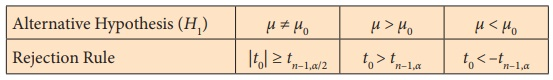### Example 2.1

The average monthly sales, based on past experience of a particular brand of tooth paste in departmental stores is Ōé╣ 200. An advertisement campaign was made by the company and then a sample of 26 departmental stores was taken at random and found that the average sales of the particular brand of tooth paste is Ōé╣ 216 with a standard deviation of Ōé╣8. Does the campaign have helped in promoting the sales of a particular brand of tooth paste?

### Solution:

Step 1 : Hypotheses

Null Hypothesis H0: ┬Ą = 200

i.e., the average monthly sales of a particular brand of tooth paste is not significantly different from Ōé╣ 200.

Alternative Hypothesis H1: ┬Ą > 200

i.e., the average monthly sales of a particular brand of tooth paste are significantly different from Ōé╣ 200. It is one-sided (right) alternative hypothesis.

Step 2 : Data

The given sample information are:

Size of the sample (n) = 26. Hence, it is a small sample.

Sample mean () = 216, Standard deviation of the sample = 8.

Step 3 : Level of significance

╬▒ = 5%

Step 4 : Test statistic

The test statistic under H0 is T =Since n is small, the sampling distribution of T is the t-distribution with (nŌĆō1) degrees of freedom.

Step 5 : Calculation of test statistic

The value of T for the given sample information is calculated fromStep 6 : Critical value

Since H1 is one-sided (right) alternative hypothesis, the critical value at ╬▒ =0.05 is

te = tn-1, ╬▒ =t25,0.05 = 1.708

Step 7 : Decision

Since it is right-tailed test, elements of critical region are defined by the rejection rule t0 >te = tn-1, ╬▒ = t25,0.05 = 1.708. For the given sample information t0 = 10.20 > te =1.708. It indicates that given sample contains sufficient evidence to reject H0. Hence, the campaign has helped in promoting the increase in sales of a particular brand of tooth paste.

### Example 2.2

A sample of 10 students from a school was selected. Their scores in a particular subject are 72, 82, 96, 85, 84, 75, 76, 93, 94 and 93. Can we support the claim that the class average scores is 90?

### Solution:

Step 1 : Hypotheses

Null Hypothesis H0: ┬Ą = 90

i.e., the class average scores is not significantly different from 90.

Alternative Hypothesis H1 : ┬Ą ŌēĀ 90

i.e., the class means scores is significantly different from 90.

It is a two-sided alternative hypothesis.

Step 2 : Data

The given sample information are

Size of the sample (n) = 10. Hence, it is a small sample.

Step 3 : Level of significance

╬▒= 5%

Step 4 : Test statistic

The test statistic under H0 is T =Since n is small, the sampling distribution of T is the t - distribution with (nŌĆō1) degrees of freedom.

Step 5 : Calculation of test statistic

The value of T for the given sample information is calculated from t0as under:Sample meanStep 6 : Critical value

Since H1 is two-sided alternative hypothesis, the critical value at ╬▒  = 0.05 is te = tn-1, ╬▒/2 = t9,0.025 = 2.262

Step 7 : Decision

Since it is two-tailed test, elements of critical region are defined by the rejection rule |t0| > te = t n-1, ╬▒/2 = t =2.262. For the given sample information |t0| = 1.806 < te = 2.262.

It indicates that given sample does not provide sufficient evidence to reject H0. Hence, we conclude that the class average scores is 90.

## 5. Test of Hypotheses for Equality of Means of Two Normal Populations (Independent Random Samples)

Procedure:

Step 1 : Let ╬╝X and ╬╝Y be respectively the means of population-1 and population-2 under study. The variances of the population-1 and population-2 are assumed to be equal and unknown given by Žā2.

Frame the null hypothesis as H0 : ┬ĄX = ┬ĄY and choose the suitable alternative hypothesis from (i) H1 : ╬╝X ŌēĀ ╬╝Y (ii) H1 : ╬╝X > ╬╝Y (iii) H1 : ╬╝X < ╬╝Y

Step 2 : Describe the sample/data. Let (X1, X2 , ŌĆ”, Xm) be a random sample of m observations drawn from Population-1 and (Y1, Y2 , ŌĆ”, Yn) be a random sample of n observations drawn from Population-2, where m and n are small (i.e., m < 30 and n < 30). Here, these two samples are assumed to be independent.

Step 3 : Set up level of significance (╬▒)

Step 4 : Consider the test statisticwhere Sp is the ŌĆ£pooledŌĆØ standard deviation (combined standard deviation) given by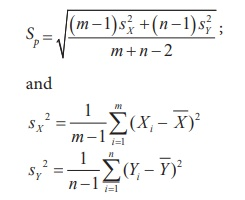The approximate sampling distribution of the test statisticis the t-distribution with m+nŌĆō2 degrees of freedom i.e., t ~ tm+nŌĆō2.

Step 5 : Calculate the value of T for the given sample ( x1 , x2 ,... xm ) and ( y1 , y 2 ,... yn ) asStep 6 : Choose the critical value, te, corresponding to ╬▒ and H1 from the following tableStep 7 : Decide on H0 choosing the suitable rejection rule from the following table corresponding to H1.Example 2.3

The following table gives the scores (out of 15) of two batches of students in an examination.Test at 1% level of significance the average performance of the students in Batch I and Batch II are equal.

Solution:

Step 1 : Hypotheses: Let ┬ĄX and ┬ĄY denote respectively the average performance of students in Batch I and Batch II. Then the null and alternative hypotheses are :

Null Hypothesis H0 : ┬Ą X = ┬ĄY

i.e., the average performance of the students in Batch I and Batch II are equal.

Alternative Hypothesis H1 : ┬Ą X ŌēĀ ┬ĄY

i.e., the average performance of the students in Batch I and Batch II are not equal.

Step 2 : Data

The given sample information are:

Sample size for Batch I : m =10

Sample size for Batch II : n = 8

Step 3 : Level of significance

╬▒= 1%

Step 4 : Test statistic

The test statistic under H0 isThe sampling distribution of T under H0 is the t-distribution with m+nŌĆō2 degrees of freedom i.e., t ~ tm+nŌĆō2

Step 5 : Calculation of test statistic

To find sample mean and sample standard deviation:To find sample means:

Let (x1 , x2 ,..., x10) and (y1, y2 ,..., y8) denote the scores of students in Batch I and Batch II respectively.To find combined sample standard deviation: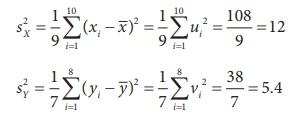Pooled standard deviation is:The value of T is calculated for the given information asStep 6 : Critical value

Since H1 is two-sided alternative hypothesis, the critical value at ╬▒ = 0.01 is te = tm+n-2, ╬▒/2 = t16,0.005 = 2.921

Step 7 : Decision

Since it is two-tailed test, elements of critical region are defined by the rejection rule |t0| < te = tm+n-2, ╬▒ = t16,0.005 = 2.921. For the given sample information |t0| = 1.3957 < te = 2.921.

It indicates that2 given sample contains insufficient evidence to reject H0. Hence, the mean performance of the students in these batches are equal.

### Example 2.4

Two types of batteries are tested for their length of life (in hours). The following data is the summary descriptive statistics.Is there any significant difference between the average life of the two batteries at 5% level of significance?

### Solution:

Step 1 : Hypotheses

Null Hypothesis H0 : ╬╝X = ╬╝Y

i.e., there is no significant difference in average life of two types of batteries A and B.

Alternative Hypothesis H0 : ╬╝X ŌēĀ ╬╝Y

i.e., there is significant difference in average life of two types of batteries A and B. It is a two-sided alternative hypothesis

Step 2 : Data

The given sample information are :

m = number of batteries under type A = 14

n = number of batteries under type B = 13= Average life (in hours) of type A battery = 94= Average life (in hours) of type B battery = 86

sX = standard deviation of type A battery =16

sY = standard deviation of type B battery = 20

Step 3 : Level of significance

╬▒= 5%

Step 4 : Test statistic

The test statistic under H0 is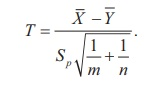The sampling distribution of T under H0 is the t-distribution with m+nŌĆō2 degrees of freedom i.e., t ~ tm+nŌĆō2

Step 5 : Calculation of test statistic

Under null hypotheses H0:where s is the pooled standard deviation given by,The value of T is calculated for the given information asStep 6 : Critical value

Since H1 is two-sided alternative hypothesis, the critical value at ╬▒ = 0.05 is te = tm+n-2, ╬▒/2 = t25, 0.025 = 2.060.

Step 7 : Decision

Since it is a two-tailed test, elements of critical region are defined by the rejection rule |t0| < te = tm+n-2,╬▒/2 = t25, 0.025 = 2.060. For the given sample information |t0| = 1.15 < te = 2.060. It indicates that2 given sample contains insufficient evidence to reject H0. Hence, there is no significant difference between the average life of the two types of batteries.

## 6. To test the equality of two means ŌĆō paired t-test

### Procedure:

Step 1 : Let X and Y be two correlated random variables having the distributions respectively N(╬╝X , ŽāX2) (Population-1) and N(╬╝Y , ŽāY2) (Population-2). Let D = XŌĆōY, then it has normal distribution N(╬╝D = ╬╝X ŌĆō ╬╝Y, ŽāD2).

Frame null hypothesis as

H0 : ╬╝D= 0

And choose alternative hypothesis from

(i) H1 : ╬╝DŌēĀ 0 (ii) H1 : ╬╝D> 0 (iii) H1 : ╬╝D< 0

Step 2 : Describe the sample/data. Let (X1, X2, ŌĆ”, Xm) be a random sample of m observations drawn from Population-1 and (Y1, Y2, ŌĆ”, Yn) be a random sample of n observations drawn from Population-2. Here, these two samples are correlated in pairs.

Step 3 : Set up level of significance (╬▒)

Step 4 : Consider the test statistic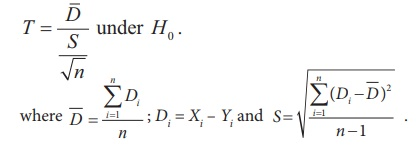The approximate sampling distribution of the test statistic T under H0 is t - distribution with (nŌĆō1) degrees of freedom.

Step 5 : Calculate the value of T for the given data asStep 6 : Choose the critical value, te, corresponding to ╬▒ and H1 from the following tableStep 7 : Decideon H0 choosing the suitable rejection rule from the following table corresponding to H1.### Example 2.5

A company gave an intensive training to its salesmen to increase the sales. A random sample of 10 salesmen was selected and the value (in lakhs of Rupees) of their sales per month, made before and after the training is recorded in the following table. Test whether there is any increase in mean sales at 5% level of significance.### Solution:

Step 1 : Hypotheses

Null Hypothesis H0 : ╬╝D= 0

i.e., there is no significant increase in the mean sales after the training.

Alternative Hypothesis H1 : ╬╝D> 0

i.e., there is significant increase in the mean sales after the training. It is a one-sided alternative hypothesis.

Step 2 : Data

Sample size n = 10

Step 3 : Level of significance

╬▒= 5%

Step 4 : Test statistic

Test statistic under the null hypothesis isThe sampling distribution of T under H0 is t - distribution with (10ŌĆō1) = 9 degrees of freedom.

Step 5 : Calculation of test statistic

To findand s:

Let x denote sales before training and y denote sales after trainingHere instead of di = xi ŌĆō yi it is assumed di = yi ŌĆō xi for calculations to be simpler.Step 6 : Critical value

Since H0 is a one-sided alternative hypothesis, the critical value at 5% level of significance is te = tn-1, ╬▒ =t9,0.05 = 1.833

Step 7 : Decision

It is a one-tailed test. Since |t0| = 1.6052 < te = tn-1, ╬▒ = t9,0.05 = 1.833, H0 is not rejected.

Hence, there is no evidence that the mean sales has increased after the training.

Tags : Properties, Procedure Steps, Example Solved Problems | Statistics , 12th Statistics : Chapter 2 : Tests Based on Sampling Distributions I
Study Material, Lecturing Notes, Assignment, Reference, Wiki description explanation, brief detail
12th Statistics : Chapter 2 : Tests Based on Sampling Distributions I : StudentŌĆÖs t Distribution and Its Applications | Properties, Procedure Steps, Example Solved Problems | Statistics